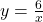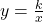Question

6=xy does this show direct variation Explain your reasoning. ANSWER THIS PLZ

1.Latifah

No. It is an inverse variation.

Step-by-step explanation:

6= xy

Making y the subject of formula:For a direct variation, the equation would be in the form of y=kx, where k is a constant.

However, the equation above is in the form of.

Thus, 6=xy does not show direct variation.